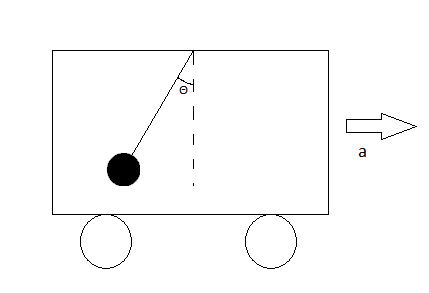# A pendulum suspended from the roof of a bus moving along a horizontal track makes an angle of 5...

## Question:

A pendulum suspended from the roof of a bus moving along a horizontal track makes an angle of {eq}5^{\circ} {/eq}with the vertical. Determine the acceleration of the bus?

## Non-Inertial Frame of Reference:

As the bus accelerates, there will be a pseudo force acting on the bob opposite to the acceleration of the bus. This force will cause the bob to make an angle with the vertical. It should be noted that the higher the acceleration of the bus, the greater will be the angle of bob with the vertical.When the bus accelerates, the bus no longer remains an inertial frame of reference. The acceleration of the bus will appear on the bob in the form of a pseudo force. The ball will experience a force opposite to the acceleration of the bus.

Thus, from the diagram, the force acting on the bob will be the gravitational force acting downwards and the pseudo force acting opposite to the acceleration of the bus.

{eq}tan\theta=\dfrac{ma}{mg}\\ tan5^{\circ}=\dfrac{a}{9.8}\\ a=0.8574\:ms^{-2} {/eq}